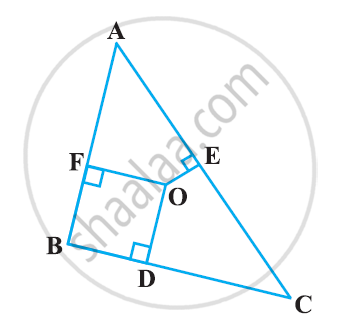# In the following figure, O is a point in the interior of a triangle ABC, OD ⊥ BC, OE ⊥ AC and OF ⊥ AB. Show that - Mathematics

In the following figure, O is a point in the interior of a triangle ABC, OD ⊥ BC, OE ⊥ AC and OF ⊥ AB. Show that(i) OA2 + OB2 + OC2 − OD2 − OE2 − OF2 = AF2 + BD2 + CE2

(ii) AF2 + BD2 + CE= AE2 + CD2 + BF2

#### Solution

(i) Applying Pythagoras theorem in ΔAOF, we obtain

OA2 = OF2 + AF2

OA2 = OF2 + AF2

Similarly, in ΔBOD

OB2 = OD2 + BD2

Similarly, in ΔCOE

OC2 = OE2 + EC2

OA2 + OB2 + OC2 = OF2 + AF2 + OD2 + BD2 + OE2 + EC2

OA2 + OB2 + OC2 – OD2 – OE2 – OF2 = AF2 + BD2 + CE2.

From above result

AF2 + BD2 + EC2 = (OA2 - OE2) + (OC2 - OD2) + (OB2 - OF2)

∴ AF2 + BD2 + CE2 = AE2 + CD2 + BF2.

Concept: Right-angled Triangles and Pythagoras Property
Is there an error in this question or solution?

#### APPEARS IN

NCERT Class 10 Maths
Chapter 6 Triangles
Exercise 6.5 | Q 8 | Page 151